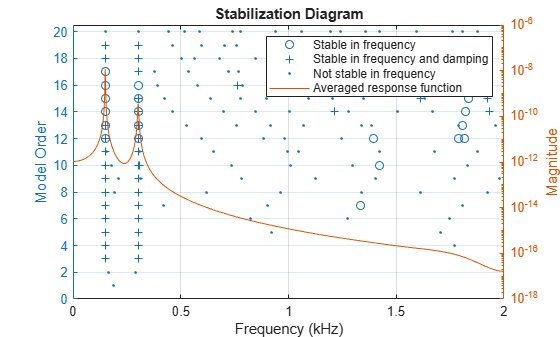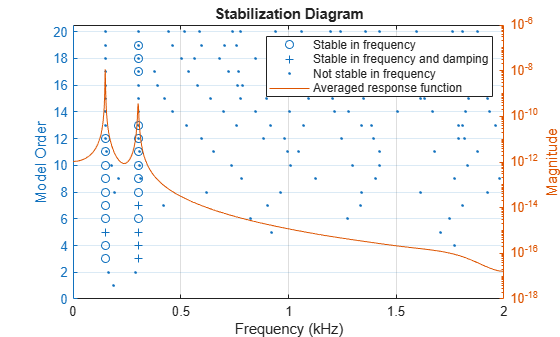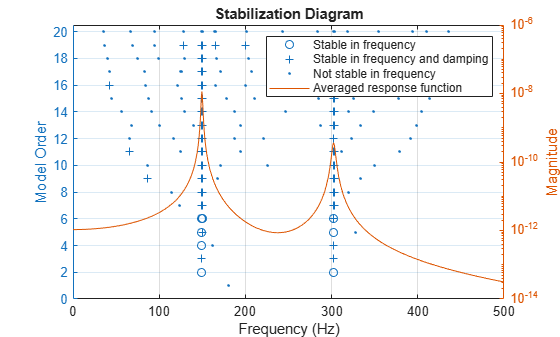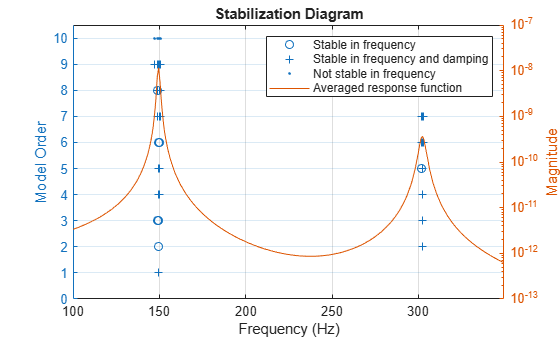# modalsd

Generate stabilization diagram for modal analysis

## Syntax

``modalsd(frf,f,fs)``
``modalsd(frf,f,fs,Name,Value)``
``fn = modalsd(___)``

## Description

````modalsd(frf,f,fs)` generates a stabilization diagram in the current figure. `modalsd` estimates the natural frequencies and damping ratios from 1 to 50 modes and generates the diagram using the least-squares complex exponential (LSCE) algorithm. `fs` is the sample rate. The frequency, `f`, is a vector with a number of elements equal to the number of rows of the frequency-response function, `frf`. You can use this diagram to differentiate between computational and physical modes.```

example

````modalsd(frf,f,fs,Name,Value)` specifies options using name-value pair arguments.```
````fn = modalsd(___)` returns a cell array of natural frequencies, `fn`, identified as being stable between consecutive model orders. The ith element contains a length-i vector of natural frequencies of stable poles. Poles that are not stable are returned as `NaN`s. This syntax accepts any combination of inputs from previous syntaxes.```

## Examples

collapse all

Compute the frequency-response functions for a two-input/two-output system excited by random noise.

Load the data file. Compute the frequency-response functions using a 5000-sample Hann window and 50% overlap between adjoining data segments. Specify that the output measurements are displacements.

```load modaldata winlen = 5000; [frf,f] = modalfrf(Xrand,Yrand,fs,hann(winlen),0.5*winlen,'Sensor','dis');```

Generate a stabilization diagram to identify up to 20 physical modes.

`modalsd(frf,f,fs,'MaxModes',20)`Repeat the computation, but now tighten the criteria for stability. Classify a given pole as stable in frequency if its natural frequency changes by less than 0.01% as the model order increases. Classify a given pole as stable in damping if the damping ratio estimate changes by less than 0.2% as the model order increases.

`modalsd(frf,f,fs,'MaxModes',20,'SCriteria',[1e-4 0.002])`Restrict the frequency range to between 0 and 500 Hz. Relax the stability criteria to 0.5% for frequency and 10% for damping.

`modalsd(frf,f,fs,'MaxModes',20,'SCriteria',[5e-3 0.1],'FreqRange',[0 500])`Repeat the computation using the least-squares rational function algorithm. Restrict the frequency range from 100 Hz to 350 Hz and identify up to 10 physical modes.

`modalsd(frf,f,fs,'MaxModes',10,'FreqRange',[100 350],'FitMethod','lsrf')`## Input Arguments

collapse all

Frequency-response functions, specified as a vector, matrix, or 3-D array. `frf` has size p-by-m-by-n, where p is the number of frequency bins, m is the number of response signals, and n is the number of excitation signals used to estimate the transfer function.

Example: `tfestimate(randn(1,1000),sin(2*pi*(1:1000)/4)+randn(1,1000)/10)` approximates the frequency response of an oscillator.

Data Types: `single` | `double`
Complex Number Support: Yes

Frequencies, specified as a vector. The number of elements of `f` must equal the number of rows of `frf`.

Data Types: `single` | `double`

Sample rate of measurement data, specified as a positive scalar expressed in hertz.

Data Types: `single` | `double`

### Name-Value Pair Arguments

Specify optional comma-separated pairs of `Name,Value` arguments. `Name` is the argument name and `Value` is the corresponding value. `Name` must appear inside quotes. You can specify several name and value pair arguments in any order as `Name1,Value1,...,NameN,ValueN`.

Example: `'MaxModes',20,'FreqRange',[0 500]` computes up to 20 physical modes and restricts the frequency range to between 0 and 500 Hz.

Fitting algorithm, specified as the comma-separated pair consisting of `'FitMethod'` and `'lsce'` or `'lsrf'`.

Frequency range, specified as the comma-separated pair consisting of `'FreqRange'` and a two-element vector of increasing, positive values contained within the range specified in `f`.

Data Types: `single` | `double`

Maximum number of modes, specified as the comma-separated pair consisting of `'MaxModes'` and a positive integer.

Data Types: `single` | `double`

Criteria to define stable natural frequencies and damping ratios between consecutive model degrees of freedom, specified as the comma-separated pair consisting of `'SCriteria'` and a two-element vector of positive values. `'SCriteria'` contains the maximum fractional differences between poles to be classified as stable. The first element of the vector applies to natural frequencies. The second element applies to damping ratios.

Data Types: `single` | `double`

## Output Arguments

collapse all

Natural frequencies identified as stable, returned as a matrix. The first i elements of the ith row contain natural frequencies. Poles that are nonphysical or not stable in frequency are returned as `NaN`s.

 Brandt, Anders. Noise and Vibration Analysis: Signal Analysis and Experimental Procedures. Chichester, UK: John Wiley & Sons, 2011.

 Ozdemir, Ahmet Arda, and Suat Gumussoy. "Transfer Function Estimation in System Identification Toolbox™ via Vector Fitting." Proceedings of the 20th World Congress of the International Federation of Automatic Control, Toulouse, France, July 2017.

 Vold, Håvard, John Crowley, and G. Thomas Rocklin. “New Ways of Estimating Frequency Response Functions.” Sound and Vibration. Vol. 18, November 1984, pp. 34–38.